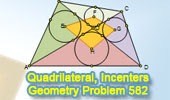## Wednesday, February 23, 2011

### Problem 582: Quadrilateral, Diagonals, Incenters, Metric Relations

Geometry Problem
Click the figure below to see the complete problem 582.Zoom at: Geometry Problem 582

1.Connect OE,OF,OG,OH
We have E,O,G collinear and F,O,H collinear ( angle bisectors of vertical angles)
We also have EG perpendicular to FH at O ( angle bisectors of 2 supplement angles)
apply Pythagoras therorem we have
EF^2+HG^2=OE^2+OF^2+OH^2+OG^2=EH^2+FG^2

Peter Tran

2.angle EOF is right angled as the bisectors of a linear pair enclose a right angle. so by applying Pythagoras theorem and associativity property of addition, we have EF^2 +GH^2 = (FO^2 + EO^2) + (HO^2 + GO^2) = (FO^2 + GO^2) + (EO^2 + HO^2) = FG^2 + EH^2.
Q. E. D.